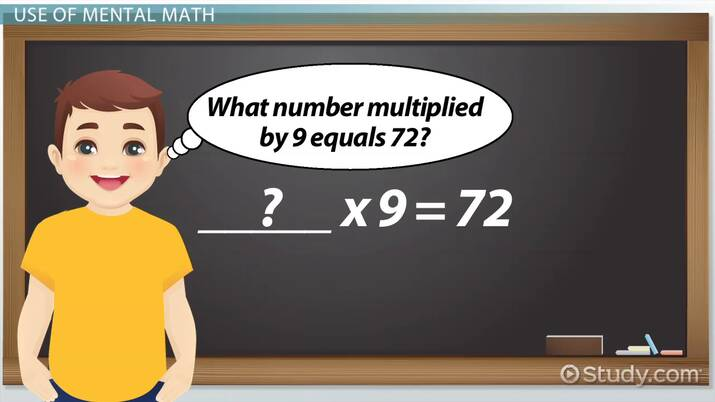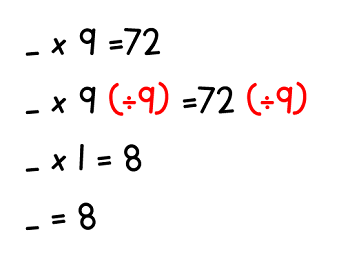# Solving Problems With More Than One Operation: Lesson for Kids

Lesson Transcript
Instructor: Mary Beth Burns

Mary Beth has taught 1st, 4th and 5th grade and has a specialist degree in Educational Leadership. She is currently an assistant principal.

Solving math problems with one operation is pretty easy, but moving on to problems with more than one operation can be just as easy. You just need to know the strategies! Updated: 05/27/2020

## Math in Real Life

Imagine that you just bought 72 brownies for a party and the baker packs the brownies in 9 boxes. If there are an equal number of brownies in each box, how many are there in each one?

Mathematically, this scenario would look like this:

_____ x 9 = 72

The blank represents the unknown number, or the number of brownies that go in each box. There are a couple of different strategies you can use to figure out what goes in the blank.An error occurred trying to load this video.

Try refreshing the page, or contact customer support.

Coming up next: Multi-Step Math Word Problems: Lesson for Kids

### You're on a roll. Keep up the good work!

Replay
Your next lesson will play in 10 seconds
• 0:04 Math in Real Life
• 0:33 Use of Mental Math
• 0:51 Use of Equations
• 1:56 PEMDAS
• 3:24 Lesson Summary
Save Save

Want to watch this again later?

Timeline
Autoplay
Autoplay
Speed Speed

## Use of Mental Math

If you've memorized your multiplication tables, you can solve this problem with mental math, which means using your brain, instead of a calculator, computer, or paper and pencil. You do this by asking yourself ''What number multiplied by 9 equals 72?'' The answer is 8.

## Use of Equations

If you haven't memorized all of your multiplication tables, you can solve this problem using an equation, a mathematical sentence that states what two numbers equal. This involves getting the unknown number on one side of the equal sign and all of the other numbers on the other side of the equation.

You can do this by performing the opposite operation on both sides of the equation. Addition and subtraction are opposite operations, as are multiplication and division. The equation must be balanced. This means that, whatever you do on one side of the equal sign, you must also do on the other side of the equal sign.To get the unknown by itself, we divide that side by 9, which leaves us with 1 x _____. Remember that, as our equation must be balanced, we have to divide the other side of the equation by 9, giving us 72 ÷ 9 = 8.

## PEMDAS

Sometimes, a math problem will involve several operations, making it difficult to know where to start. But don't worry, the order of operations can serve as a guide for solving multi-step equations.

Parentheses

Exponents

Multiplication,

To unlock this lesson you must be a Study.com Member.

### Register to view this lesson

Are you a student or a teacher?